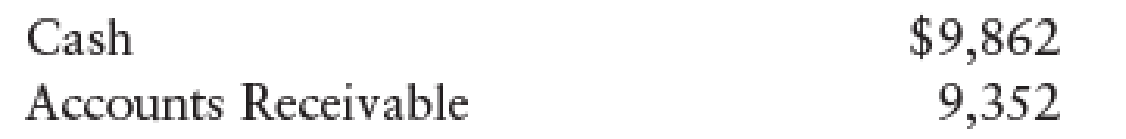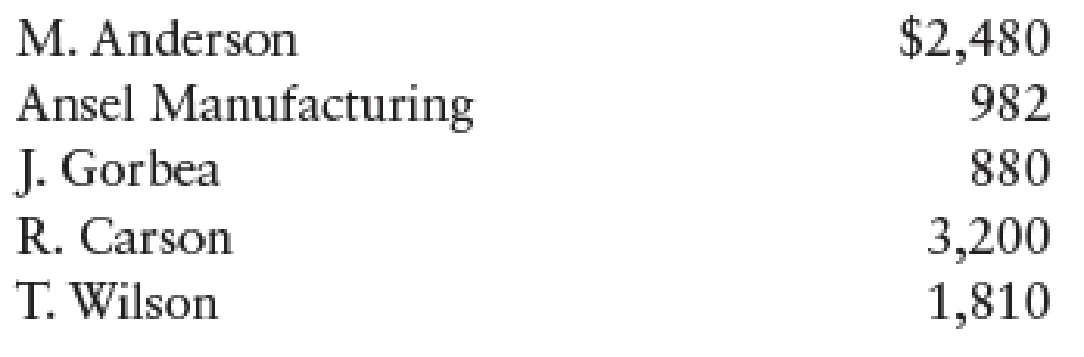Chapter 12, Problem 7SPA

Chapter
Section
Textbook Problem

CASH RECEIPTS JOURNAL Zebra Imaginarium, a retail business, had the following cash receipts during December 20--. The sales tax is 6%.Dec. 1    Received payment on account from Michael Anderson, $1,360.2 Received payment on account from Ansel Manufacturing,$382.7    Made cash sales for the week, $3,160, plus tax. Bank credit card sales for the week,$1,000, plus tax. Bank credit card fee is 3%.Dec. 8    Received payment on account from J. Gorbea, $880.11 Michael Anderson returned merchandise for a credit,$60, plus tax.14    Made cash sales for the week, $2,800, plus tax. Bank credit card sales for the week,$800, plus tax. Bank credit card fee is 3%.20    Received payment on account from Tom Wilson, $1,110.21 Ansel Manufacturing returned merchandise for a credit,$22, plus tax.21    Made cash sales for the week, $3,200, plus tax.24 Received payment on account from Rachel Carson,$2,000.Beginning general ledger account balances were as follows:Beginning customer account balances were as follows:Required 1. Record the transactions in the cash receipts journal. Total and verify column totals and rule the columns. Use the general journal to record sales returns and allowances. 2. Post from the journals to the general ledger and accounts receivable ledger accounts. Use account numbers as shown in the chapter.

1.

To determine

Prepare the given transactions in the cash receipts journal and verify the total column and rule the column and use the general journal to record the sales returns and allowances.

Explanation

Cash Receipts Journal:  It is a special book where only cash receipts transactions that are received from customers, merchandise sales and service made in cash and collection of accounts receivable are recorded.

The following are the some examples of transactions that would be recorded in the Other Accounts credit column of the cash receipts journal:

• • Cash received as interest on notes payable
• • Interest revenue received from debtors
• • Cash receipts from bank loans
• • Cash receipts for capital investments

Prepare the given transactions in the cash receipts journal and verify the total column and rule the column and use the general journal to record the sales returns and allowances:

Table (1)

Verification of total debit and credit column:

Total Debit     = Total Credit($17,292.36+$57.24)=($5,732.00+$10,960.00+$657.60)=$17,349.6=$17,349.6 Working note 1: Calculate the amount of cash on dated 7th December: Cash(debit)on dated 7thDecember}=(Cash sales + 6% on sales tax)=[$3,160+(6%×$3,160)]=[$3,160+$189.60]=$3,349.60

Working note 2:

Calculate the amount of bank credit card expense on dated 7th December:

Bank credit card expense on dated 7thDecember}=[(Sales + 6% on sales tax)×Credit card fee %]=[$1,000+(6%×$1,000)]×3%=[$1,000+$60]×3%=$31.80 Working note 3: Calculate the amount of cash on dated 7th December: Cash(debit)on dated 7thDecember}=[(Cash sales + 6% on sales tax)Bank credit card expense]=[$1,000+(6%×$1,000)$31.80]=[$1,000+$60$31.80]=$1,028.20

Working note 4:

Calculate the amount of cash on dated 14th December:

Cash(debit)on dated 14thDecember}=(Cash sales + 6% on sales tax)=[$2,800+(6%×$2,800)]=[$2,800+$168]=$2,968 Working note 5: Calculate the amount of bank credit card expense on dated 14th December: Bank credit card expense on dated 14thDecember}=[(Sales + 6% on sales tax)×Credit card fee %]=[$800+(6%×$800)]×3%=[$800+$48]×3%=$25

2.

To determine

Post the prepared journal to the general ledger, and to the accounts receivable ledger.

Still sussing out bartleby?

Check out a sample textbook solution.

See a sample solution

The Solution to Your Study Problems

Bartleby provides explanations to thousands of textbook problems written by our experts, many with advanced degrees!

Get Started